# Two-balloon experiment explained

15 July 2015
Masa Sakano

This document is written in the MathML format. Most of modern web-browsers should be able to deal with it. But if you find a problem in browsing equations etc, you may want to have a look at the PDF file instead. Also, 日本語版 (Japanese version) is available.

## 1. Quiz

There are two balloons. You inflate them to different sizes, bigger and smaller. Now, what will happen if you connect the mouths of those two balloons? Guess!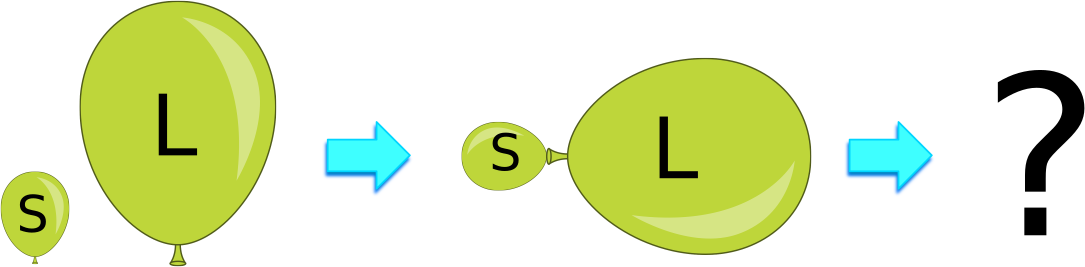Figure 1: Quiz: What will happen when two balloons inflated to different sizes are connected?

This is a relatively famous problem called Two-balloon experiment''. The result is actually a little counter-intuitive.

In this article I explain what happens and why, first based on the law of physics in Section 2, and then present more intuitive interpretation without using equations in Section 3.

## 2. Mathematical solution

### 2.1 Balloon surface tension with Hook's law

#### 2.1.1 Calculations

Suppose there is a perfectly spherical balloon with a rubber with the initial radius of ${r}_{0}$, that is, when there is no tension on the rubber (Left panel in Fig.2). There is no excess pressure over the atmospheric pressure of 1 atm in the air inside the balloon at this stage.

The amount of the tension in rubber for a certain stretch is proportional to the amount of material of the rubber. Providing the density of the rubber is uniform, and providing the thickness of a sheet of it is uniform (and negligibly small), it is proportional to the area of the rubber. Let us define $k$ as a spring constant for a certain original area and shape of the rubber. Hook's law states that the force of a tension $F$, when a (ideal, namely linearly elastic) spring is stretched by the length $x$, is $F=kx$, using the spring constant $k$.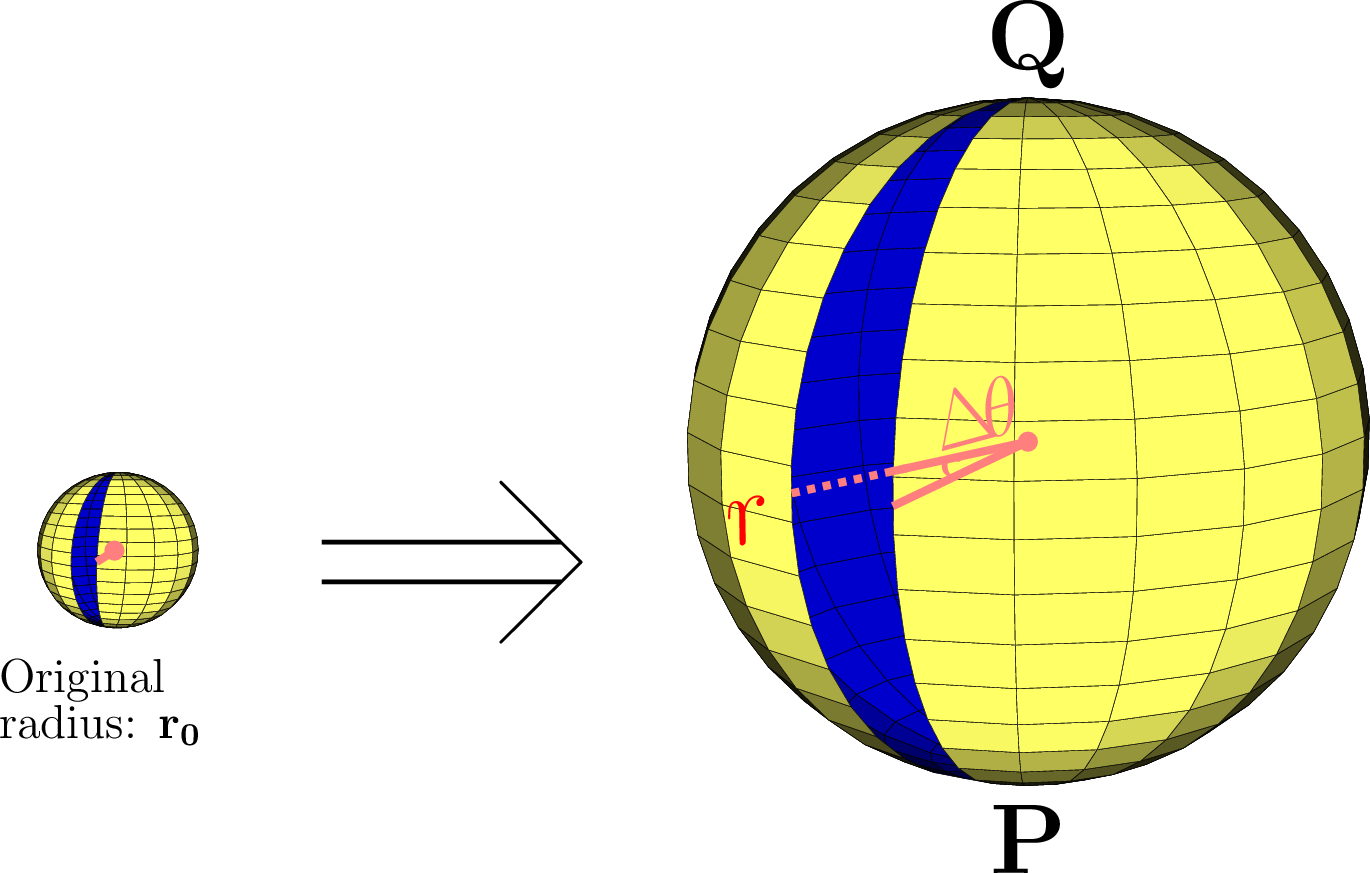Figure 2: A balloon with the initial radius of (Left) ${r}_{0}$ is inflated to (Right) $r$. we consider the narrow strip of the rubber $\overline{PQ}$ (blue area) with the viewing angle of $\Delta \theta$.

Then, if a very narrow strip of the rubber ( $\overline{PQ}$ in Fig.2), of which the viewing angle from the origin of the coordinates is $\Delta \theta$, is stretched to the circular length of $\pi r$ (with the radius $r$) from the original length of $\pi {r}_{0}$, keeping the perfect spherical profile, the tension force $F\left(r,\Delta \theta \right)$ between $\overline{PQ}$ is, $\begin{array}{cccc}\phantom{\rule{6.0em}{0ex}}& \hfill F\left(r,\Delta \theta \right)& \propto k\left(\pi r-\pi {r}_{0}\right)\genfrac{}{}{0.1ex}{}{\Delta \theta }{2\pi }\hfill & \hfill \text{}\phantom{\rule{6.0em}{0ex}}\\ \hfill & =Ak\left(r-{r}_{0}\right)\Delta \theta ,\hfill & \hfill \text{(1)}\phantom{\rule{6.0em}{0ex}}\end{array}$ where $A$ is a non-dimensional constant. Note that the exact value of $A$ is not quite trivial to calculate, and is not discussed here, however it does not affect the argument below.

We now consider the balance of the works by between the rubber tension and (excess) pressure of the air inside, when this balloon of rubber is stretched from the radius of $r$ by an infinitesimal amount of $\Delta r$ to $r+\Delta r$, as it is inflated. The work ( ${W}_{p}$) by the excess pressure ( $P\left(r\right)$) of the air inside over the atmospheric air pressure for the given radius $r$ of the balloon is written as, $\begin{array}{cccc}\phantom{\rule{6.0em}{0ex}}& \hfill {W}_{p}& =P\left(r\right)\genfrac{}{}{0.1ex}{}{4\pi }{3}\left\{{\left(r+\Delta r\right)}^{3}-{r}^{3}\right\}\hfill & \hfill \text{(2)}\phantom{\rule{6.0em}{0ex}}\\ \hfill & \approx 4\pi {r}^{2}\Delta rP\left(r\right).\hfill & \hfill \text{(3)}\phantom{\rule{6.0em}{0ex}}\end{array}$ Each of tiny strips of the rubber for the angle of $\Delta \theta$ does a work $\Delta {W}_{b}$ of, using its force (Eq. 1), $\begin{array}{cccccc}\phantom{\rule{6.0em}{0ex}}& \hfill \Delta {W}_{b}& =F\left(r,\Delta \theta \right)\pi \Delta r\hfill & \phantom{\rule{6em}{0ex}}& \hfill & \hfill \text{(4)}\phantom{\rule{6.0em}{0ex}}\\ \hfill & =Ak\left(\pi r-\pi {r}_{0}\right)\genfrac{}{}{0.1ex}{}{\Delta \theta }{2\pi }\pi \Delta r\hfill & \hfill & \hfill \text{}\phantom{\rule{6.0em}{0ex}}\\ \hfill & =\genfrac{}{}{0.1ex}{}{A\pi k}{2}\left(r-{r}_{0}\right)\Delta r\Delta \theta \hfill & \hfill \left(r\ge {r}_{0}\right).& \hfill \text{(5)}\phantom{\rule{6.0em}{0ex}}\end{array}$ Therefore, the work ${W}_{b}$ by the rubber of the entire balloon is obtained by integrating this $\Delta {W}_{b}$ (Eq. 5) over $2\pi$ radians as, $\begin{array}{cccccc}\phantom{\rule{6.0em}{0ex}}& \hfill {W}_{b}& =\underset{0}{\overset{2\pi }{\int }}\genfrac{}{}{0.1ex}{}{A\pi k}{2}\left(r-{r}_{0}\right)\Delta rd\theta \hfill & \phantom{\rule{6em}{0ex}}& \hfill & \hfill \text{(6)}\phantom{\rule{6.0em}{0ex}}\\ \hfill & =A{\pi }^{2}k\left(r-{r}_{0}\right)\Delta r\hfill & \hfill \left(r\ge {r}_{0}\right).& \hfill \text{(7)}\phantom{\rule{6.0em}{0ex}}\end{array}$ Since both the works, ${W}_{p}$ (Eq.3) and ${W}_{b}$ (Eq.7), must balance out, it gives, $\begin{array}{cccccc}\phantom{\rule{6.0em}{0ex}}& \hfill 4\pi {r}^{2}\Delta rP\left(r\right)& =A{\pi }^{2}k\left(r-{r}_{0}\right)\Delta r\hfill & \phantom{\rule{6em}{0ex}}& \hfill & \hfill \text{(8)}\phantom{\rule{6.0em}{0ex}}\\ \hfill \therefore \phantom{\rule{0.278em}{0ex}}\phantom{\rule{0.278em}{0ex}}P\left(r\right)& =\genfrac{}{}{0.1ex}{}{A\pi k}{4}\genfrac{}{}{0.1ex}{}{r-{r}_{0}}{{r}^{2}}\hfill & \hfill & \hfill \text{}\phantom{\rule{6.0em}{0ex}}\\ \hfill & =\genfrac{}{}{0.1ex}{}{A\pi k}{4}\genfrac{}{}{0.1ex}{}{1}{r}\left(1-\genfrac{}{}{0.1ex}{}{{r}_{0}}{r}\right)\hfill & \hfill \left(r\ge {r}_{0}\right)& \hfill \text{(9)}\phantom{\rule{6.0em}{0ex}}\\ \hfill & \propto \left\{\begin{array}{cc}-{r}_{0}{\left(\genfrac{}{}{0.1ex}{}{1}{r}-\genfrac{}{}{0.1ex}{}{1}{2{r}_{0}}\right)}^{2}+\genfrac{}{}{0.1ex}{}{1}{4{r}_{0}}\hfill & \left(r\sim {r}_{0}\right)\hfill \\ \genfrac{}{}{0.1ex}{}{1}{r}\hfill & \left(r\gg {r}_{0}\right),\hfill \end{array}\phantom{\right\}}\hfill & \hfill & \hfill \text{(10)}\phantom{\rule{6.0em}{0ex}}\end{array}$ as shown in Fig.3.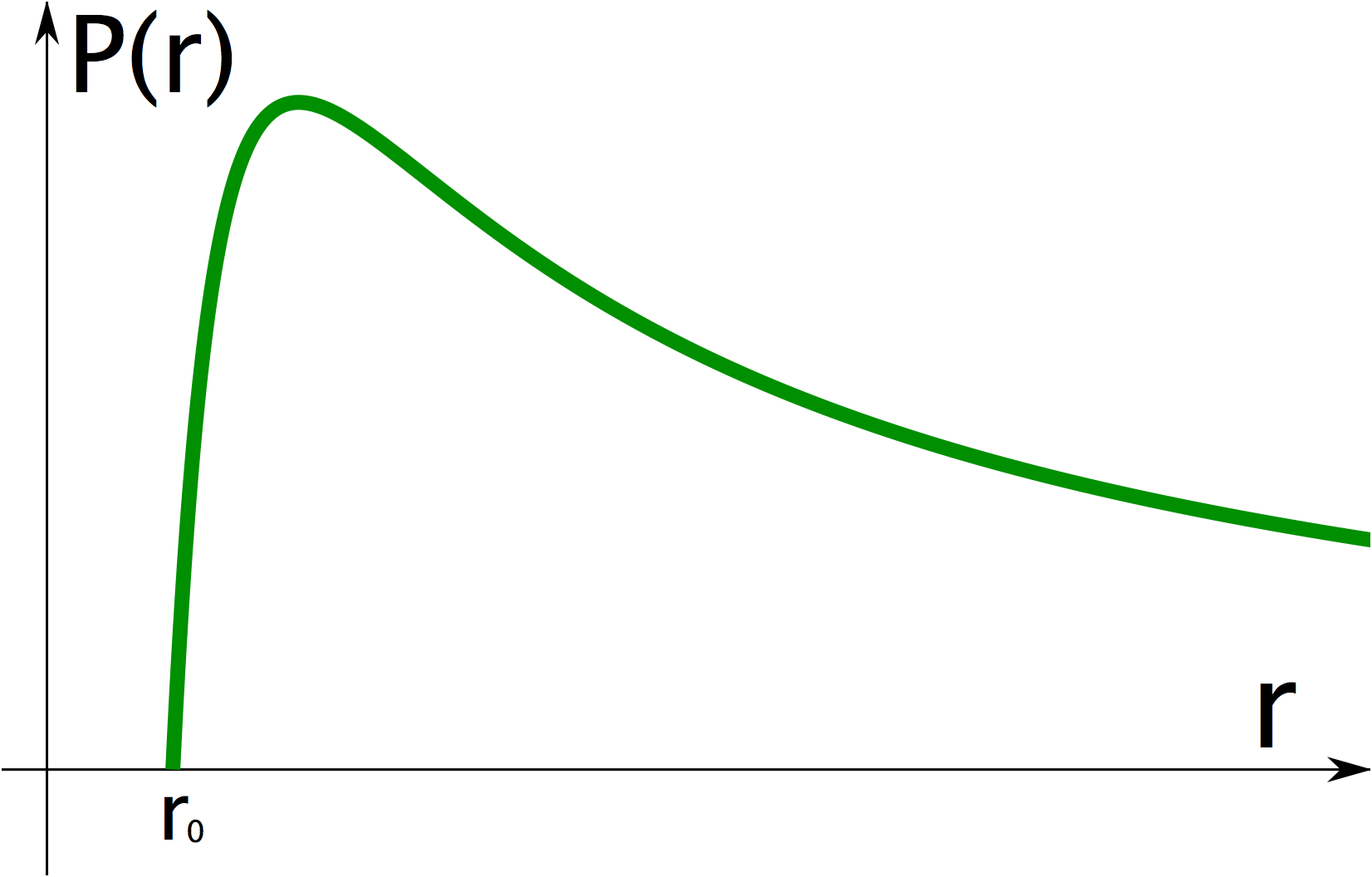Figure 3: Air pressure inside a spherical balloon as a function of the radius $r$.

Consequently, the air pressure inside the balloon

1. sharply increases as a function of the radius $r$ to a peak, then sharply decreases beyond,
2. decreases inversely proportional to the radius $r$, where $r$ is far greater than ${r}_{0}$.

Note that the above argument is not exact. In the more strict formulation, based on the James-Guth stress-strain relation, the first part of the equation, which is dominant at $r\sim {r}_{0}$, is inversely proportional to the negative ${r}^{6}$  with a certain offset, as opposed to ${r}^{2}$ as derived above. Namely, the shapes of the functions between the strict one and the derived one here are broadly similar, but the former is a much more steep function of $r$. On the other hand, the second part, which is dominant at a larger $r$, is the same, and is inversely proportional to $r$.

Another note is that Hook's law is a good approximation in general for rubber, but only when the rubber is not exceedingly stretched. It is known the surface tension acutely increases again when the rubber is stretched close to its physical maximum limit of stretch, that is, before the breakage.

#### 2.1.2 Direct interpretation

When the two balloons are connected, the equilibrium will be achieved so that the air pressure inside, which is in equilibrium with the surface tension of the balloon, is uniform in both the balloons.

The above formulation states that once the radius of a balloon exceeds a threshold, which is fairly close to the original radius (roughly 38 per cent larger than the original ), bigger the balloon is, smaller the pressure is. Thus, providing both the balloons are inflated to the size bigger than the threshold, the air pressure of the smaller one is larger than that of the bigger one. Therefore, once two balloons are connected, the smaller balloon pushes out some of its air, while shrinking, to the bigger one, which becomes even bigger. Then a positive feedback kicks in to accelerate this move, because the air pressure of the smaller and bigger balloon becomes even higher and lower, respectively, until they reach the equilibrium when the radius of the smaller balloon decreases somewhere below the threshold and hence the air pressures in both the balloons become the same.

This equilibrium point is stable, because of the difference in the function shapes (Eq.10) at $r>{r}_{0}$ and $r\gg {r}_{0}$ for the smaller and bigger balloons, respectively. For any small perturbation from the equilibrium point, the negative feedback works, and the equilibrium will be restored quickly.

Note that there is another equilibrium point in this configuration, i.e., the sizes of both the balloons, hence the air pressures inside, are the same. One could force to achieve this configuration by pressing down the bigger balloon. However, this equilibrium is unstable. Any perturbation induces a positive feedback, and then the more stable equilibrium as mentioned above will be achieved quickly.

As noted in the previous subsection, if a balloon is inflated exceedingly beyond a certain point, its air pressure sharply increases again as a function of $r$. In that case that the air pressure of the bigger balloon is already higher than that of the smaller balloon, the bigger balloon will become smaller, transferring a part of its air to the smaller balloon to the equilibrium point, where the pressures of the two balloons are equal.

#### 2.1.3 An intuitive interpretation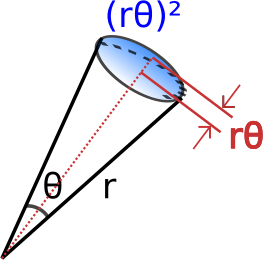Figure 4: Pressure by the rubber at the surface to the air inside the cone.

Let's think of a cone from the centre of a balloon to its surface. The air inside is pressed by the surface rubber towards the centre, namely the pressure. The amount of the force ( ${F}_{r}$) exerted to the area is proportional to the length of stretch of the rubber along the radial direction, which is proportional to the radius of the balloon, $r$. Intuitively, you can think of the length between the plane of the feet of those cone surface and the centre of the surface in the radial direction, which is $\approx r{\theta }^{2}$, and accordingly ${F}_{r}\propto r{\theta }^{2}$ (See Fig.4). The surface area $S$, to which the force is exerted to, is proportional to the square of the radius $r$, which is $S\approx \genfrac{}{}{0.1ex}{}{{\left(r\theta \right)}^{2}}{2}$. Therefore the pressure is $\genfrac{}{}{0.1ex}{}{{F}_{r}}{S}\propto \genfrac{}{}{0.1ex}{}{2}{r}\propto {r}^{-1}$.

Consequently, the pressure is inversely proportional to the radius (when the radius is large enough).

### 2.2 Volume and surface-area relation

Another way to understand the two-balloon problem is from the point of view of the total surface area and volume. In balloon, more surface area means a more stretch in rubber, which requires more energy (or potential). For a given volume of the air inside, a sphere is the best shape to maintain the smallest surface area for it. For example, for a volume of 1 litre, the total surface areas are $\sim$484 cm2 and 600 cm2 for a sphere and a square cube, respectively. Therefore, balloons are almost spherical (or elliptic) in shape, and never have a jagged surface.

Now, suppose the total volume of the air for the two balloon combined is $V$. After the two balloons are connected, what is the most efficient ratio ( $a$) of the radii between the two balloons to keep the volume, yet achieving the least surface area, hence the least surface tension? The total volume $V$ is written as, $\begin{array}{ccc}\phantom{\rule{6.0em}{0ex}}& V=\genfrac{}{}{0.1ex}{}{4\pi }{3}\left(1+{a}^{3}\right){r}^{3}\phantom{\rule{0.167em}{0ex}}\phantom{\rule{0.167em}{0ex}}\phantom{\rule{0.167em}{0ex}}\left(a\ge 0\right).\hfill & \hfill \text{(11)}\phantom{\rule{6.0em}{0ex}}\end{array}$ The total surface area $S$ of the two balloons are, $\begin{array}{cccc}\phantom{\rule{6.0em}{0ex}}& \hfill S& =4\pi \left(1+{a}^{2}\right){r}^{2}\hfill & \hfill \text{(12)}\phantom{\rule{6.0em}{0ex}}\\ \hfill & ={\left\{9\left(4\pi \right){V}^{2}\right\}}^{\genfrac{}{}{0.1ex}{}{1}{3}}{\left(1+{a}^{2}\right)}^{\genfrac{}{}{0.1ex}{}{1}{3}}\phantom{\rule{0.167em}{0ex}}\phantom{\rule{0.167em}{0ex}}\phantom{\rule{0.167em}{0ex}}\left(a\ge 0\right).\hfill & \hfill \text{(13)}\phantom{\rule{6.0em}{0ex}}\end{array}$ Therefore, the surface area becomes the smallest when $a=0$, namely, the radius of a balloon is 0.

In reality, the surface tension of the rubber of a balloon drops to 0 at the original radius ( ${r}_{0}$ in Section 2.1.1), which is small but not 0. Also, there is a further slight deviation in the actual balloon from this way of thinking, as mentioned previously. But this is one of intuitive approximations in thinking over this quiz, where the sizes of both the balloons are much bigger than the original.

## 3. Intuitive explanation

Seeing is believing --- have a look at a video of the actual experiment :

As you see, after the two balloons in different sizes are connected, the smaller balloon becomes even smaller and the bigger one becomes bigger.

Why?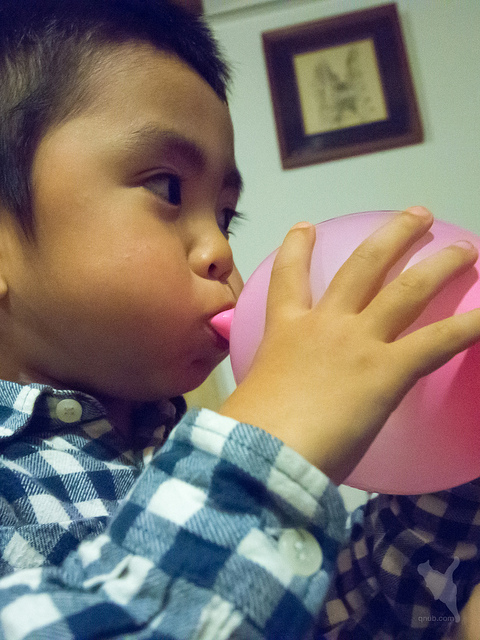Figure 5: You need the best effort when starting inflating a balloon. Photo courtesy [©Norihiro Kataoka]

In short it is because smaller a balloon is, higher the air pressure inside is, except when the size of the balloon is very close to the original (uninflated) one or to its physical limit of breakage. So, once the two balloons are connected, a part of the air in the smaller balloon is pushed to the bigger balloon. This in turn shrinks the smaller balloon further and increases the air pressure even more, while the bigger balloon becomes even bigger and its air pressure decreases even more. Then this move will continue, until the smaller balloon becomes nearly the original size, and the (rubber of) smaller balloon does not give the air inside the extra pressure.

To remind you of this nature of balloons, think of the following. Do you remember what happens why you try to inflate a balloon by blowing up with your own breath? It is the hardest, or it needs the best effort, at the initial stage of blowing up. Once it passes the stage and a balloon is inflated a little, the further inflation is much easier.

Or, you can make a little experiment. Blow up a balloon big. Hold the balloon (at the mouth part) so that it won't shoot off to the air uncontrollably. Then, Release the air from the mouth, while feeling the air pressure from the mouth with the palm of the other hand. You will notice the air pressure will increase quickly as the balloon shrinks more and more.

They are clear demonstrations of the nature of balloons. It is not counter-intuitive if you think of these cases!

Now, you understand balloons do have this characteristics. Then what would happen when two balloons are connected is its natural result.

The next question may be why so.

The pressure is a force spread over an area. So, bigger the area is, smaller the pressure is. If you want to pierce solid food, like an undercooked potato, you know it would be a lot easier with a skewer or fork than a point of your finger. That is because the surface area of a skewer and fork is a lot smaller than a finger, and so you would need a less force to do the job.

Now, let's think of a part of a balloon, a cone with curved bottom (See Fig.4 for it, ignoring the letters). The air pressure inside the cone was caused by the stretch of the rubber outwards from the centre. Bigger the balloon is, more the rubber stretches, and more the force will be. However, at the same time, the surface area will become bigger, much more than the outward stretch of the rubber, and accordingly, much more than the increase of the force by the rubber.

For example, if the size (or radius or diameter) of the balloon becomes doubled, the force by the rubber will be doubled, but the surface area will be 4 times as big, so the pressure will be only a half. If the size becomes tripled, the force will be tripled, but the surface area will be 9 times as big, so the pressure will be only one-third.

That is why bigger a balloon is, lower the air pressure is. So, if you connect two balloons with different sizes, the air pressure of the smaller balloon beat the bigger one's. The smaller balloon will become even smaller and the bigger balloon will become even bigger.